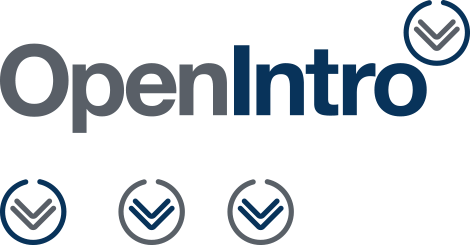Select to expand
Select to collapse

# Course ePortfolio

## Introduction to Statistics

This collection contains the California Open Educational Resources Council (CA OER) list of peer-reviewed textbooks for Introduction to Statistics (common course-id MATH 110), one of the top 50 college courses identified for inclusion in the California Open Online Library for Education (COOLforEd).

CID Number:  MATH 110

General Course Description:  MATH 110

The use of probability techniques, hypothesis testing, and predictive techniques to facilitate decision-making. Topics include descriptive statistics; probability and sampling distributions; statistical inference; correlation and linear regression; analysis of variance, chi-square and t-tests; and application of technology for statistical analysis including the interpretation of the relevance of the statistical findings.  Applications using data from disciplines including business, social sciences, psychology, life science, health science, and education.

Minimum Units: 3.0

Any rationale or comment:  Typically satisfies general education quantitative reasoning requirement (CSU GE B4).

Share

### Prerequisites

Prerequisite(s): Intermediate Algebra

Corequisite(s): None

### Pedagogical Approach & Learning Outcomes

None

#### Learning Outcomes

Upon successful completion of the course, students will be able to:

• Distinguish among different scales of measurement and their implications;
• Interpret data displayed in tables and graphically;
• Apply concepts of sample space and probability;
• Calculate measures of central tendency and variation for a given data set;
• Identify the standard methods of obtaining data and identify advantages and disadvantages of each;
• Calculate the mean and variance of a discrete distribution;
• Calculate probabilities using normal and student’s t-distributions;
• Distinguish the difference between sample and population distributions and analyze the role played by the Central Limit Theorem;
• Construct and interpret confidence intervals;
• Determine and interpret levels of statistical significance including p-values;
• Interpret the output of a technology-based statistical analysis;
• Identify the basic concept of hypothesis testing including Type I and II errors;
• Formulate hypothesis tests involving samples from one and two populations;
• Select the appropriate technique for testing a hypothesis and interpret the result;
• Use linear regression and ANOVA analysis for estimation and inference, and interpret the associated statistics; and
• Use appropriate statistical techniques to analyze and interpret applications based on data from disciplines including business, social sciences, psychology, life science, health science, and education.

### Assessment & Other Information

#### Assessment

• Tests
• Examinations
• Homework or projects where students demonstrate their mastery of the learning objectives and their ability to devise, organize and present complete solutions to problems.

None

### Course Resources

•Introductory Statistics (Open (Access) Textbook)
The Shafer and Zhang open textbooks is designed as a one-semester statistics course for general education students. The... More
•Introductory Statistics (Open (Access) Textbook)
The Illowsky and Dean one-semester open textbook is geared towards students not majoring in math and science and focuses... More
•Developed by Rice University, University of Houston at Clear Lake, and Tufts University, this open textbook is a public... More
•OpenIntro Statistics, 3rd Edition (Open (Access) Textbook)
We hope readers will take away three ideas from this book in addition to forming a foundation of statistical thinking... More